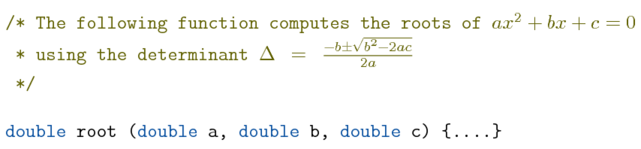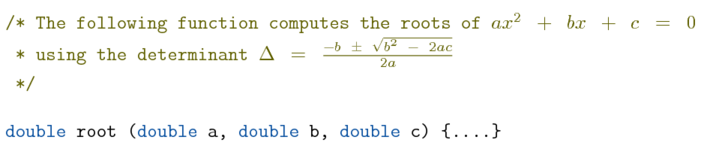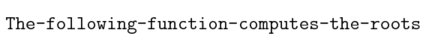# Correct math escape in t-vim

Posted on July 22, 2017

There is a feature in t-vim module that allows the use of TeX code in comments, which is useful when typeset math in comments. For example:

\definevimtyping[C][syntax=c, escape=on]

\startC
/* The following function computes the roots of \m{ax^2+bx+c=0}
* using the determinant \m{\Delta=\frac{-b\pm\sqrt{b^2-2ac}}{2a}}
*/

double root (double a, double b, double c) {....}
\stopC


The escape=on option activates this feature. Only \, {, and } have their usual meaning inside the Comment region, so I use \m{...} to enter math mode. The above code get typeset as:Gerion Entrup reported on the context mailing list that the spacing inside the math mode is not always correct. The incorrect behavior is not visit in the above example, because there was no blank space inside the math mode. As soon as we add space in the math mode, the output is too spaced out. For example,

\definevimtyping[C][syntax=c, escape=on]

\startC
/* The following function computes the roots of \m{ax^2 + bx + c = 0}
* using the determinant \m{\Delta = \frac{-b \pm \sqrt{b^2 - 2ac}}{2a}}
*/

double root (double a, double b, double c) {....}
\stopCSpacing inside math mode should not affect the output. What is happening here? After a bit of sleuthing, I found the culprit.

As I had ranted in an old blog post, I want syntax highlighting programs to generate clean TeX output. Therefore, I do not escape space and newline characters. After all, it is easy enough to tell ConTeXt to honor spaces and newlines by using \obeyspaces and \obeylines. By themselves, \obeyspaces and \obeylines are okay.

\bgroup
\obeylines\obeyspaces\tttf
The following function computes the roots of \m{ax^2 + bx + c = 0}
using the determinant \m{\Delta = \frac{-b \pm \sqrt{b^2 - 2ac}}{2a}}
\egroupIn t-vim, I wanted to control how to wrap long lines. The default option is not to do anything and let the user figure out how to wrap the source code. There is also an option to split a long line at a space. (For other options on splitting long lines, see the documentation).

To control whether a long line should be split at a space or not, I redefined \obeyedspace. For those who are not familiar with ConTeXt internals, whenever \obeyspaces is active, space is mapped to \obeyedspace. This makes it possible, for example, to visualize spaces. For example,

\bgroup
\obeylines\obeyspaces\tttf\def\obeyedspace{-}
The following function computes the roots
\egroupSo, to allow a line to break at a space, I use

\def\obeyedspace{\hskip\interwordspace\relax}


and to prevent lines from breaking at a space, I use

\def\obeyedspace{\kern\interwordspace\relax}


However, this definition creates a wreck inside math mode.

\bgroup
\obeylines\obeyspaces\tttf
\def\obeyedspace{\hskip\interwordspace\relax}
The following function computes the roots of \m{ax^2 + bx + c = 0}
using the determinant \m{\Delta = \frac{-b \pm \sqrt{b^2 - 2ac}}{2a}}
\egroupNow, that we know what is going on, it is an easy fix (suggested by Henri Menke). Define \obeyedspace to

\def\obeyedspace{\mathortext\normalspace{\hskip\interwordspace\relax}}


Let’s test this.

\bgroup
\obeylines\obeyspaces\tttf
\def\obeyedspace{\mathortext\normalspace{\hskip\interwordspace\relax}}
The following function computes the roots of \m{ax^2 + bx + c = 0}
using the determinant \m{\Delta = \frac{-b \pm \sqrt{b^2 - 2ac}}{2a}}
\egroupThis bug has been fixed in t-vim version 2017.07.29

This entry was posted in T-Vim and tagged math, horizontal spacing, code formatting.